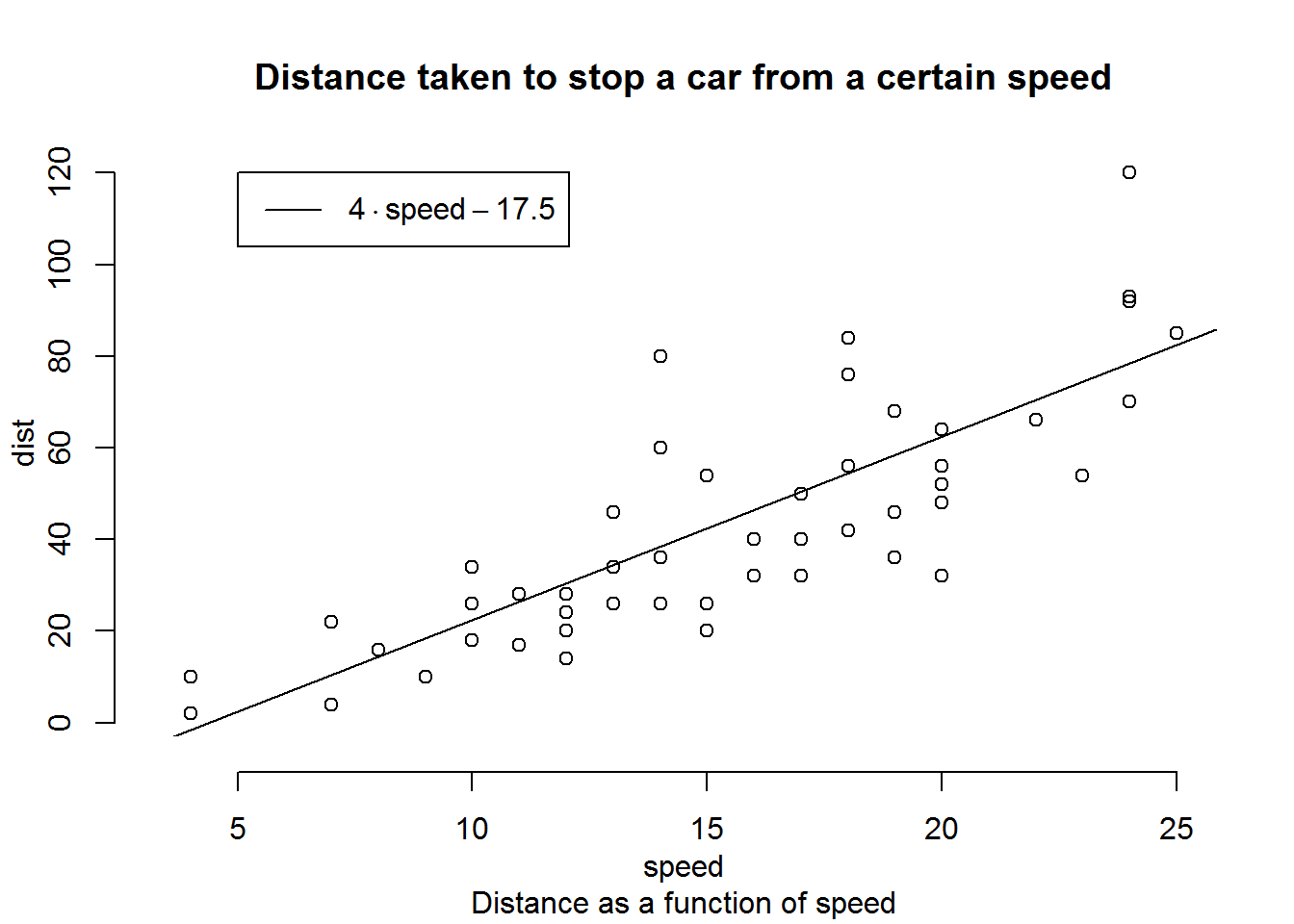### QUESTION 08

## Topic: Sheet 6, Exercise 5

## Question: The text in the legend is generated with the commend expression()". Although I could not use expression(). I used the following commend: "legend(5,120, legend = "4·Speed-17.5", lty = 1)". How can I use expression()?

data(cars)
# plot(cars$speed, cars$dist, axes = FALSE, xlab = "", ylab = "")
# abline(-17.5, 4)
# axis(side = 1, at = seq(from = 5, to = 25, by=5), line = 1)
# axis(side = 2, at = seq(from = 0, to = 120, by = 20), line = 1)
# legend(5,120, legend = "4Â·Speed-17.5", lty = 1)
# title(main = "Distance taken to stop a car from a certain speed", sub = "Distance as a function of speed", xlab = "speed", ylab = "dist")

## Answer: In the argument legend of the function legend() you may use function expression() to generate the legend.

plot(cars$speed, cars$dist, axes = FALSE, xlab = "", ylab = "")
abline(-17.5, 4)
axis(side = 1, at = seq(from = 5, to = 25, by=5), line = 1)
axis(side = 2, at = seq(from = 0, to = 120, by = 20), line = 1)
title(main = "Distance taken to stop a car from a certain speed", sub = "Distance as a function of speed", xlab = "speed", ylab = "dist")
legend(5,120, legend = expression(4%.%speed-17.5), lty = 1)# Note that the argument is interpreted as a mathematical expression and the output will be formatted according to TeX-like rules. In this example the syntax "x %.% y" means   "x dot y"